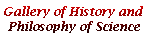Pierre de Fermat

One of the legendary mathematicians. He published little, but devised many problems which challenged many mathematicians after him. His reputation was established through correspondence with various people, including such eminent figures as Descartes, Mersenne, Pascal, and Huygens. He was a lawyer, and lived in Toulouse mostly.

His name is associated with various problems of mathematics, geometry, calculus, optics, and probability, for instance. But by far the most important is the number theory. His famous "Last Theorem" claims (x^n signifies the nth power of x):

x^n+y^n=z^n

has no non-zero integer solutions for x, y and z when nÅÑ 2.

Many mathematicians seeked to find a correct proof for this claim, but it was only in 1994 that a British mathematician (Andrew Wiles) succeeded in its proof, drawing on many results by predecessors.

BACK TO FERMAT PICTURE